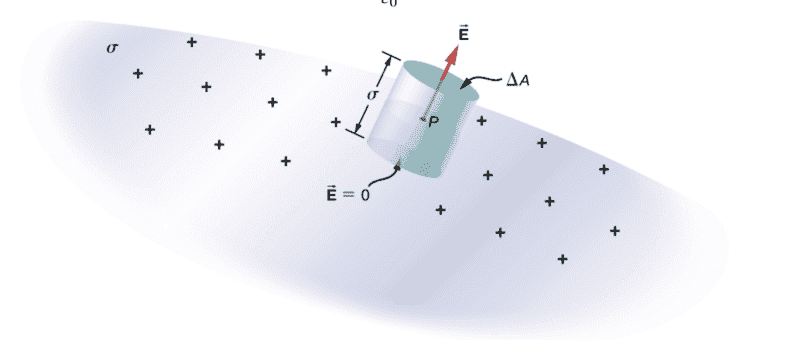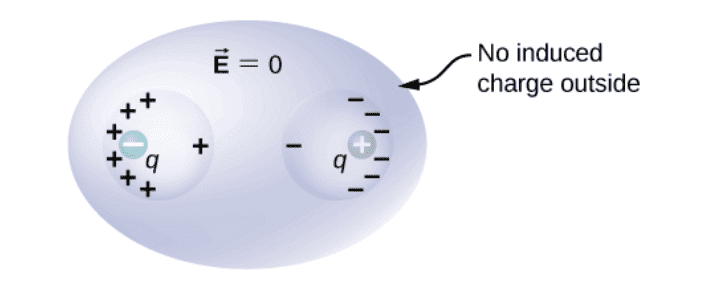# Electric field, flux, and conductor questions

• I
• annamal
I thought the electric field canceled out. Is that correct?No, the electric field does not cancel out. The electric field is always there, just not as strong.f

#### annamal

1) Why is the electric field 0 at the bottom of Gaussian surface? Isn't the electric field on both sides of the surface, pointing down and outwards like a plane of charge? see image.2) Why does a charge distribution with cylindrical symmetry have to be infinitely long?
3) My book says a positive charge outside the conductor induces only the free electrons to move, so there are no positive charges that are actually moving? The excess electrons creates a negative side and the devoid of electrons creates a positive side so no positive charges are actually being repulsed away?
4) If I put a positive rod inside the cavity of a conductor and touch it inside, then take it away, does the rod retain being positive or did the electrons induced in the inside cavity move to the rod?
5) Is the net electric field of a charge 0 because the radial field lines cancel each other due to symmetry?
6) Why is there no induced charge outside of the conductor with positive and negative charges in a conductor's cavity? See image•Delta2
As for 1), by the attached figure I observe that the bottom side is within the closed surface of charge and the top side is outward. If it is so, considering another closed surface where the bottom side is on that contains no charge inside, E=0 at the bottom side.

As for 1), by the attached figure I observe that the bottom side is within the closed surface of charge and the top side is outward. If it is so, considering another closed surface where the bottom side is on that contains no charge inside, E=0 at the bottom side.
Yes, I see that but the surface charges have electric fields radiating outwards...so don't know why it doesn't have an electric field pointing down

Yes, I see that but the surface charges have electric fields radiating outwards...so don't know why it doesn't have an electric field pointing down
What does Gauss' theorem tell you about the field inside a hollow spherical shell of uniform charge density?

What does Gauss' theorem tell you about the field inside a hollow spherical shell of uniform charge density?
I think I get it, but my explanation is the electric field lines radiating into the sphere all cancel out leaving no electric field inside the sphere

I think I get it, but my explanation is the electric field lines radiating into the sphere all cancel out leaving no electric field inside the sphere
You are correct that there is no field, but if there is no field then there are no field lines. That's why none are shown.

You are correct that there is no field, but if there is no field then there are no field lines. That's why none are shown.
Do you know the answers to my other question or should I create different threads for them?

1) Why is the electric field 0 at the bottom of Gaussian surface? Isn't the electric field on both sides of the surface, pointing down and outwards like a plane of charge? see image.
View attachment 300479
2) Why does a charge distribution with cylindrical symmetry have to be infinitely long?
3) My book says a positive charge outside the conductor induces only the free electrons to move, so there are no positive charges that are actually moving? The excess electrons creates a negative side and the devoid of electrons creates a positive side so no positive charges are actually being repulsed away?
4) If I put a positive rod inside the cavity of a conductor and touch it inside, then take it away, does the rod retain being positive or did the electrons induced in the inside cavity move to the rod?
5) Is the net electric field of a charge 0 because the radial field lines cancel each other due to symmetry?
6) Why is there no induced charge outside of the conductor with positive and negative charges in a conductor's cavity? See image
View attachment 300480
UPDATE: 1, 2, 3, and 5 are answered.
I just need answers to 4 and 6.

for 4) yes if the rod is conductor too then the two conductors, because we touch them, have to be at the same potential , so some of the positive charge from rod has to move to the conductor, or equivalently some free electrons from the conductor to the positive rod. So the positive rod will have smaller positive charge after we touch them.

for 4) yes if the rod is conductor too then the two conductors, because we touch them, have to be at the same potential , so some of the positive charge from rod has to move to the conductor, or equivalently some free electrons from the conductor to the positive rod. So the positive rod will have smaller positive charge after we touch them.
I didn't know the positive charges could move. I thought only the free electrons were moving.

I wonder why the positive rod is not completely neutralized.

I didn't know the positive charges could move. I thought only the free electrons were moving.

I wonder why the positive rod is not completely neutralized.
You are right positive charges can't move, so free electrons move in order to equalize the potential. If the positive rod was completely neutralized that would mean that its potential would be zero, and so the conductor potential would be zero, so both conductor and rod at zero potential, which also would mean they have zero total charge, which violates conservation of charge, because the total charge at the start wasn't zero.

You are right positive charges can't move, so free electrons move in order to equalize the potential. If the positive rod was completely neutralized that would mean that its potential would be zero, and so the conductor potential would be zero, so both conductor and rod at zero potential, which also would mean they have zero total charge, which violates conservation of charge, because the total charge at the start wasn't zero.
No, the conductor we touched would have net positive charge on the outside and the rod would have neutral charge.

No, the conductor we touched would have net positive charge on the outside and the rod would have neutral charge.
if this was the case then the conductor and rod wouldn't be at same potential.

Basically when we touch them their potential becomes the same and we can conclude everything else from this fact. It is not that their charge becomes the same or that one charge becomes zero.

Last edited:
if this was the case then the conductor and rod wouldn't be at same potential.

Basically when we touch them their potential becomes the same and we can conclude everything else from this fact. It is not that their charge becomes the same or that one charge becomes zero.
And the rod being less positive would create the same potential?

And the rod being less positive would create the same potential?
It will not have the same potential as before we touch but it will have the same potential with the conductor.

The potential of something does not depend only on the charge it carries but on its shape/geometry too.